# De Sitter space

complete space-like submanifolds in a

Let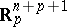be an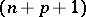-dimensional Minkowski space of index, i.e.,and is equipped with the Lorentz metric. For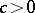, let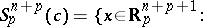Thus,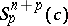is an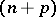-dimensional indefinite Riemannian manifold of indexand of constant curvature. It is called an-dimensional de Sitter space of constant curvatureand of index. E. Calabi, S.Y. Cheng and S.T. Yau proved that a complete maximal space-like hypersurface in a Minkowski spacepossesses a remarkable Bernstein property. As a generalization of the Bernstein-type problem, S. Ishihara proved that a complete maximal space-like submanifold in a de Sitter spaceis totally geodesic (cf. Totally-geodesic manifold). It was proved by K. Akutagawa [a1], Q.M. Cheng [a2] and K.G. Ramanathan that complete space-like submanifolds with parallel mean curvature vector in a de Sitter spaceare totally umbilical (cf. also Differential geometry) if

1)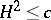, when;

2), when. The conditions 1) and 2) are best possible. When, Akutagawa and Ramanathan constructed many examples of space-like submanifolds in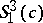that are not totally umbilical. When,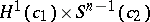, where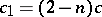and, is a complete space-like hypersurface inof constant mean curvaturethat is not totally umbilical and satisfies. Cheng gave a characterization of complete non-compact hypersurfaces inwith: a complete non-compact hypersurface in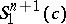with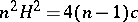is either isometric toor its Ricci curvature is positive and the squared norm of the second fundamental form is a subharmonic function. Therefore, the Cheeger–Gromoll splitting theorem implies that a complete non-compact hypersurfaceinwithis isometric toif the number of its ends is not less than. S. Montiel [a4] has proved that a compact space-like hypersurface inof constant mean curvature is totally umbilical, and Aiyama has generalized this to compact space-like submanifolds inwith parallel mean curvature vector and flat normal bundle. Complete space-like hypersurfaces inwith constant mean curvature have also been characterized under conditions on the squared norm of the second fundamental form.

Cf. also Anti-de Sitter space.